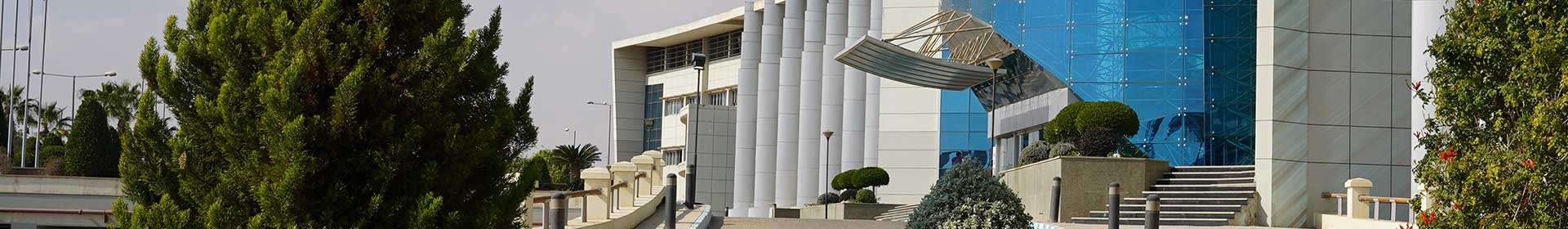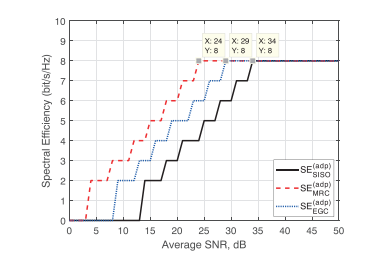Journal

# Spectral efficiency improvement with SISO and SIMO in M-QAM over millimeter-wave links

By
Al-Nahhal M.
Ismail T.
Nasr M.E.

This paper proposes a spectral efficiency improvement technique for millimeter wave (mmWave) links. The proposed technique provides an efficient utilization of the mmWave link capacity. This technique is applied in three cases the single-input single-output (SISO), single-input multiple-output (SIMO) with the maximal ratio combining and with the equal gain combining. The M-ary quadrature amplitude modulation scheme is used in our work. The power series expansion is used for deriving closed-form expressions for bit error rate (BER) performances in all studied cases. The BER closed-form expressions are confirmed by the numerical solution of the integral equations. The simulation results show that a high spectral efficiency can be accomplished by the proposed technique. As well as the derived expressions closely match with the numerical solution of integration expressions at different values of modulations order the Rician factor. For instance, the spectral efficiency gain achievement is 8 at signal-to-noise ratio (SNR) equals 34 dB in the case of SISO system whereas in the case of SIMO system, the same gain is achieved at SNR equals 24 dB. As well as the BER performance is enhanced from 1.188 × 10 −4 , 7.112 × 10 −4 , 4.164 × 10 −3 , and 3.286 × 10 −2 to 8.717 × 10 −16 , 1.119 × 10 −12 , 1.308 × 10 −9 , and 4.905 × 10 −6 for M = 4, 16, 64, and 256, respectively, at SNR equals 30 dB. © 2019 John Wiley & Sons, Ltd.